Work, Energy, and Power - Lesson 1 - Basic Terminology and Concepts

PowerThe quantity work has to do with a force causing a displacement. Work has nothing to do with the amount of time that this force acts to cause the displacement. Sometimes, the work is done very quickly and other times the work is done rather slowly. For example, a rock climber takes an abnormally long time to elevate her body up a few meters along the side of a cliff. On the other hand, a trail hiker (who selects the easier path up the mountain) might elevate her body a few meters in a short amount of time. The two people might do the same amount of work, yet the hiker does the work in considerably less time than the rock climber. The quantity that has to do with the rate at which a certain amount of work is done is known as the power. The hiker has a greater power rating than the rock climber.

Power is the rate at which work is done. It is the work/time ratio. Mathematically, it is computed using the following equation.

Power = Work / time

or

P = W / t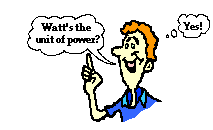The standard metric unit of power is the Watt. As is implied by the equation for power, a unit of power is equivalent to a unit of work divided by a unit of time. Thus, a Watt is equivalent to a Joule/second. For historical reasons, the horsepower is occasionally used to describe the power delivered by a machine. One horsepower is equivalent to approximately 750 Watts.

Most machines are designed and built to do work on objects. All machines are typically described by a power rating. The power rating indicates the rate at which that machine can do work upon other objects. Thus, the power of a machine is the work/time ratio for that particularmachine. A car engine is an example of a machine that is given a power rating. The power rating relates to how rapidly the car can accelerate the car. Suppose that a 40-horsepower engine could accelerate the car from 0 mi/hr to 60 mi/hr in 16 seconds. If this were the case, then a car with four times the horsepower could do the same amount of work in one-fourth the time. That is, a 160-horsepower engine could accelerate the same car from 0 mi/hr to 60 mi/hr in 4 seconds. The point is that for the same amount of work, power and time are inversely proportional. The power equation suggests that a more powerful engine can do the same amount of work in less time.

A person is also a machine that has a power rating. Some people are more power-full than others. That is, some people are capable of doing the same amount of work in less time or more work in the same amount of time. A common physics lab involves quickly climbing a flight of stairs and using mass, height and time information to determine a student's personal power. Despite the diagonal motion along the staircase, it is often assumed that the horizontal motion is constant and all the force from the steps is used to elevate the student upward at a constant speed. Thus, the weight of the student is equal to the force that does the work on the student and the height of the staircase is the upward displacement.Suppose that Ben Pumpiniron elevates his 80-kg body up the 2.0-meter stairwell in 1.8 seconds. If this were the case, then we could calculate Ben's power rating. It can be assumed that Ben must apply an 800-Newton downward force upon the stairs to elevate his body. By so doing, the stairs would push upward on Ben's body with just enough force to lift his body up the stairs. It can also be assumed that the angle between the force of the stairs on Ben and Ben's displacement is 0 degrees. With these two approximations, Ben's power rating could be determined as shown below.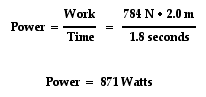Ben's power rating is 871 Watts. He is quite a horse.

Another Formula for Power

The expression for power is work/time. And since the expression for work is force*displacement, the expression for power can be rewritten as (force*displacement)/time. Since the expression for velocity is displacement/time, the expression for power can be rewritten once more as force*velocity. This is shown below.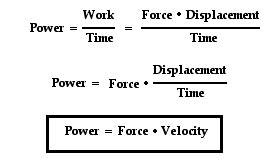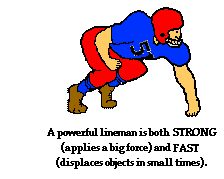This new equation for power reveals that a powerful machine is both strong (big force) and fast (big velocity). A powerful car engine is strong and fast. A powerful piece of farm equipment is strong and fast. A powerful weightlifter is strong and fast. A powerful lineman on a football team is strong and fast. A machine that is strong enough to apply a big force to cause a displacement in a small mount of time (i.e., a big velocity) is a powerful machine.

Use your understanding of work and power to answer the following questions. When finished, click the button to view the answers.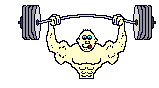1. Two physics students, Will N. Andable and Ben Pumpiniron, are in the weightlifting room. Will lifts the 100-pound barbell over his head 10 times in one minute; Ben lifts the 100-pound barbell over his head 10 times in 10 seconds. Which student does the most work? ______________ Which student delivers the most power? ______________ Explain your answers.

2. During a physics lab, Jack and Jill ran up a hill. Jack is twice as massive as Jill; yet Jill ascends the same distance in half the time. Who did the most work? ______________ Who delivered the most power? ______________ Explain your answers.3. A tired squirrel (mass of approximately 1 kg) does push-ups by applying a force to elevate its center-of-mass by 5 cm in order to do a mere 0.50 Joule of work. If the tired squirrel does all this work in 2 seconds, then determine its power.4. When doing a chin-up, a physics student lifts her 42.0-kg body a distance of 0.25 meters in 2 seconds. What is the power delivered by the student's biceps?

5. Your household's monthly electric bill is often expressed in kilowatt-hours. One kilowatt-hour is the amount of energy delivered by the flow of l kilowatt of electricity for one hour. Use conversion factors to show how many joules of energy you get when you buy 1 kilowatt-hour of electricity.

6. An escalator is used to move 20 passengers every minute from the first floor of a department store to the second. The second floor is located 5.20 meters above the first floor. The average passenger's mass is 54.9 kg. Determine the power requirement of the escalator in order to move this number of passengers in this amount of time.